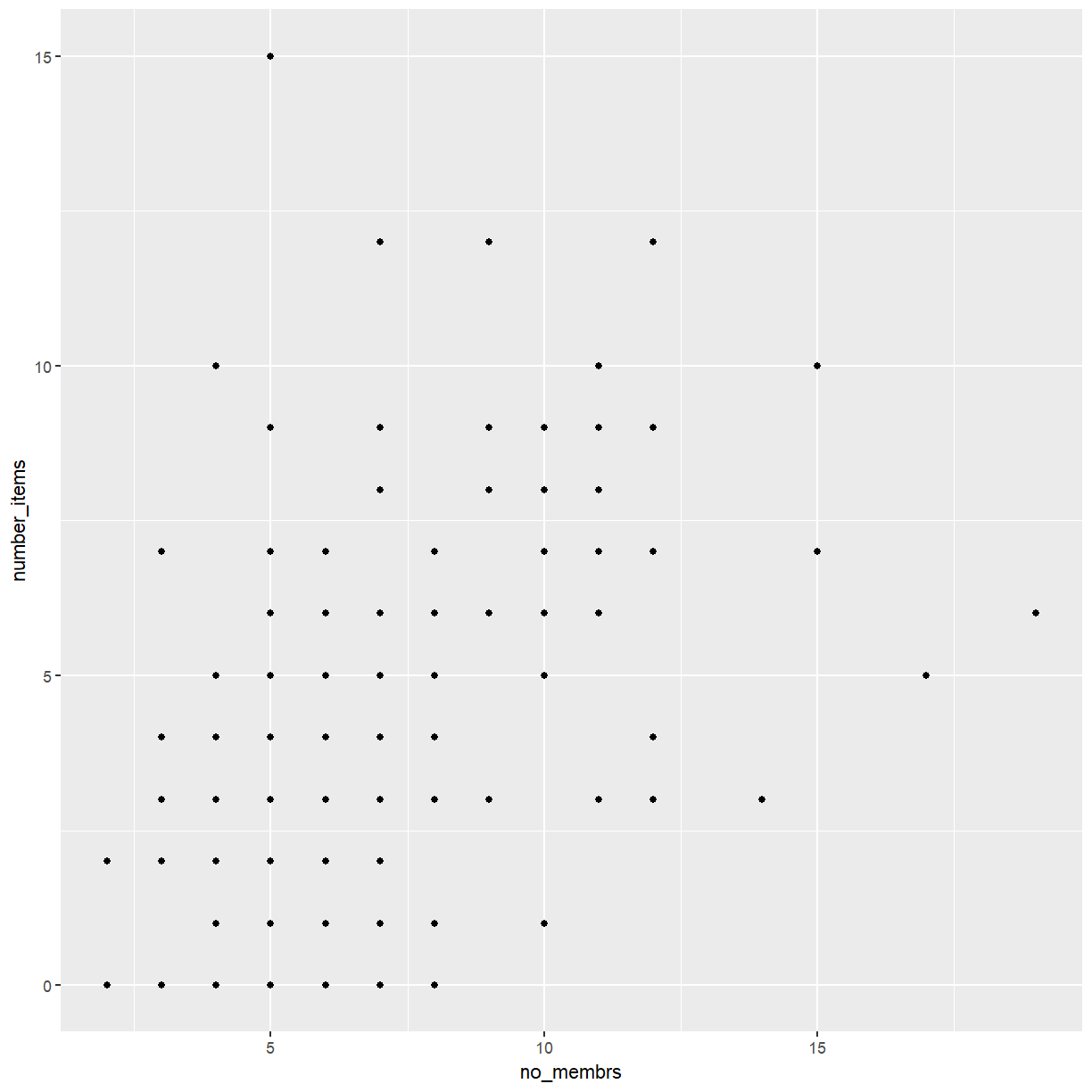This lesson is being piloted (Beta version)

# Visualising continuous data with ggplot2

## Overview

Teaching: 60 min
Exercises: 30 min
Questions
• What are the components of a ggplot?

• How do I create histograms, density plots, and scatterplots?

• How can I change the aesthetics (ex. colour, transparency) of my plot?

Objectives
• Produce histograms, density plots and scatterplots using ggplot.

• Set universal plot settings.

We start by loading the required package. `ggplot2` is also included in the `tidyverse` package.

``````library(tidyverse)
``````

The data is available as part of the project you cloned from GitHub during the previous lesson. Switch to that project now if you haven’t opened it in RStudio already.

``````interviews_plotting <- read_csv("data_output/interviews_plotting.csv")
``````
``````Parsed with column specification:
cols(
.default = col_logical(),
key_ID = col_integer(),
village = col_character(),
interview_date = col_datetime(format = ""),
no_membrs = col_integer(),
years_liv = col_integer(),
respondent_wall_type = col_character(),
rooms = col_integer(),
memb_assoc = col_character(),
affect_conflicts = col_character(),
liv_count = col_integer(),
items_owned = col_character(),
no_meals = col_integer(),
months_lack_food = col_character(),
instanceID = col_character(),
number_month_lack_food = col_integer(),
number_items = col_integer()
)
``````
``````See spec(...) for full column specifications.
``````

Before we proceed to plot the data, let’s take a quick look at what we just loaded.

``````View(interviews_plotting)
``````

## Plotting with `ggplot2`

`ggplot2` is a plotting package that makes it simple to create complex plots from data stored in a data frame. It provides a programmatic interface for specifying what variables to plot, how they are displayed, and general visual properties. Therefore, we only need minimal changes if the underlying data change or if we decide to change from a bar plot to a scatterplot. This helps in creating publication quality plots with minimal amounts of adjustments and tweaking.

`ggplot2` functions like data in the ‘long’ format, i.e., a column for every dimension, and a row for every observation. Well-structured data will save you lots of time when making figures with `ggplot2`

ggplot graphics are built step by step by adding new elements. Adding layers in this fashion allows for extensive flexibility and customization of plots.

To build a ggplot, we will use the following basic template that can be used for different types of plots:

``````ggplot(data = <DATA>, mapping = aes(<MAPPINGS>)) +  <GEOM_FUNCTION>()
``````
• use the `ggplot()` function and bind the plot to a specific data frame using the `data` argument
``````ggplot(data = interviews_plotting)
``````
• define a mapping (using the aesthetic (`aes`) function), by selecting the variables to be plotted and specifying how to present them in the graph, e.g. as x/y positions or characteristics such as size, shape, color, etc.

## Exercise

We would like to inspect the data for a potential relationship between the number of people living in a household (`no_members`) and the number of items owned by that household (`number_items`). What would be a good way to visualise these data?

## Solution

Since we are dealing with two continuous variables, a scatterplot seems like a good starting point. However, both of these variables only take a relatively small number of discrete values. Under these circumstances a scatterplot may be problematic. Let’s take a look.

``````ggplot(data = interviews_plotting, aes(x = no_membrs, y = number_items))
``````
• add ‘geoms’ – graphical representations of the data in the plot (points, lines, bars). `ggplot2` offers many different geoms; we will use some common ones today, including:

• `geom_point()` for scatter plots, dot plots, etc.
• `geom_boxplot()` for, well, boxplots!
• `geom_line()` for trend lines, time series, etc.

To add a geom to the plot use the `+` operator. To create a scatterplot we want the data to be represented as points, which can be achieved with `geom_point()`:

``````ggplot(data = interviews_plotting, aes(x = no_membrs, y = number_items)) +
geom_point()
``````The `+` in the `ggplot2` package is particularly useful because it allows you to modify existing `ggplot` objects. This means you can easily set up plot templates and conveniently explore different types of plots, so the above plot can also be generated with code like this:

``````# Assign plot to a variable
interviews_plot <- ggplot(data = interviews_plotting, aes(x = no_membrs, y = number_items))

# Draw the plot
interviews_plot +
geom_point()
``````
• Anything you put in the `ggplot()` function can be seen by any geom layers that you add (i.e., these are universal plot settings). This includes the x- and y-axis mapping you set up in `aes()`.
• You can also specify mappings for a given geom independently of the mapping defined globally in the `ggplot()` function.
• The `+` sign used to add new layers must be placed at the end of the line containing the previous layer. If, instead, the `+` sign is added at the beginning of the line containing the new layer, `ggplot2` will not add the new layer and will return an error message.